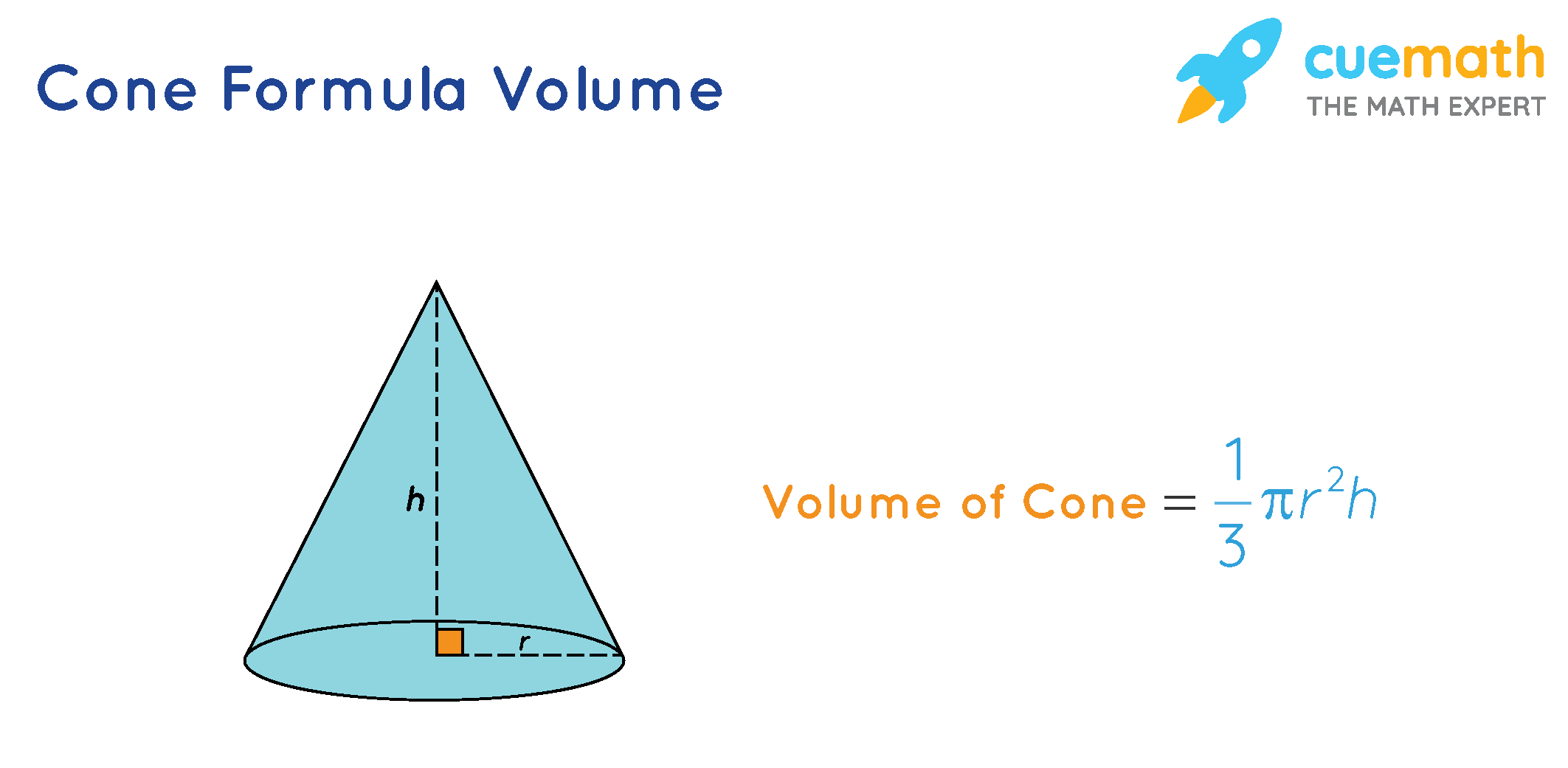# Cone Formula Volume

Go back to  'Math Formulas'

A cone is a three-dimensional figure with one circular base and a vertex.
The volume of a cone is the amount of space it occupies or we can say that the volume denotes the capacity of the cone.
The volume of a cone is measured in cubic units (in3, ft3, cm3, m3, etc.).

## What is Cone Formula Volume?

Cone Formula Volume of a given value can be expressed as,

V=1/3(Area of the base × height of the cone), or

V=1/3πr2h

Note: The formula  V=1/3πr2h is the same for all types of cone weather for an oblique cone or for a right cone.Let us have a look at a few solved examples to understand the cone formula volume better.

## Solved Examples Using Cone Formula Volume

### Jack is filling a conical bag with gems and wants to measure the capacity of each bag. The dimensions of the bag are such that the radius is 3 inches and the height is 8 inches. Find the volume of the conical bag.

Solution:

To find: Volume of the conical bag

The given dimensions are:

Radius = 3 inch, and height = 8 inch

Using Cone Formula Volume,

The volume of a cone (V)=1/3πr2h

Substituting the values  The volume of a cone=1/3×π×32×8

= 24π in3

### Jill went to a circus. On his way back home, a clown gifted him a conical cap. He measured the dimensions of a cap and observed that the height was 13 inches and the radius was 18 inches. Find the volume of the cap.

Solution:

To find: The volume of the cap

Given dimensions are: